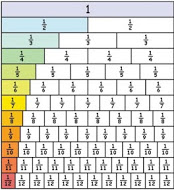Egyptian fractions are unit fractions, that is fractions with a numerator of 1. We are going to investigate methods of reducing all fractions to the sums of different smaller unit fractions as we see in this example:$\frac{5}{12}= \frac{1}{3}+\frac{1}{12}\\ \frac{3}{4}=\frac{1}{2}+\frac{1}{4}...$

Try some for yourself. Check your answers using a Fraction Wall. Does this always work? Could you explain to someone else how to use this method?

For a fraction $\frac{a}{b}$ find the largest unit fraction smaller than $\frac{a}{b}$ and subtract it. Repeat this process until you get a unit fraction. For example $\frac{1}{3}<\frac{3}{7}<\frac{1}{2}$ and $\frac{3}{7}-\frac{1}{3}=\frac{2}{21}$

$\frac{1}{11}<\frac{2}{21}<\frac{1}{10}$ and $\frac{2}{21}-\frac{1}{11}=\frac{1}{231}$  so

$\frac{3}{7}=\frac{1}{3} + \frac{1}{11}+\frac{1}{231}$

However, there are different ways to reduce unit fractions to the sums of different smaller unit fractions. For example $\frac{1}{6}= \frac{1}{7}+\frac{1}{42}\\ \frac{1}{6}=\frac{1}{8}+\frac{1}{24}\\ \frac{1}{6}=\frac{1}{9}+\frac{1}{18}\\ \frac{1}{6}=\frac{1}{10}+\frac{1}{15}\\ \frac{1}{6}=\frac{1}{11}+\frac{1}{14}+\frac{1}{231}....$

Can all unit fractions be made up in more than one way like this? Why? Try some examples of your own to test out your ideas.

Tagged with: